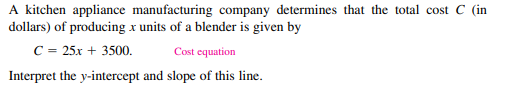### ¿Todavía tienes preguntas de matemáticas?

Pregunte a nuestros tutores expertos
Algebra
PreguntaA kitchen appliance manufacturing company determines that the total cost $$C$$ (in dollars) of producing $$x$$ units of a blender is given by

$$C = 25 x + 3500$$ . Cost equation

Interpret the $$y$$ -intercept and slope of this line.

y-intercept = 3500

slope = 25

The y-intercept tells the overhead cost when no units of the blender are produced

The slope tells the cost factor when 1 unit of blender is produced.

Solución
View full explanation on CameraMath App.